# Analyze the Frames shown below. Draw the shear and moment diagrams and sketch the approximate deflected...

Analyze the Frames shown below. Draw the shear and moment diagrams and sketch the approximate deflected shape

Settlement at A = 1” and at C = .5” E = 30000 kis, I = 240 in4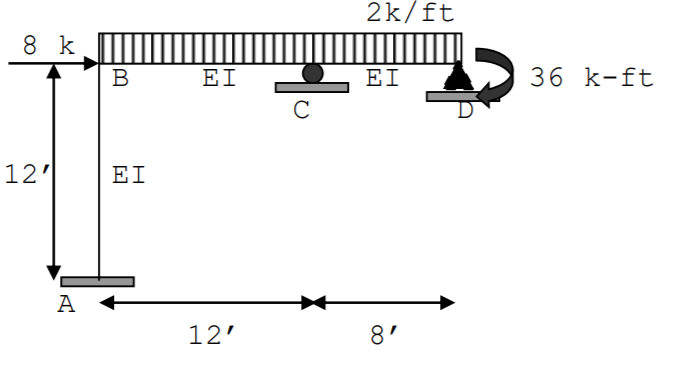2k/ft 8 k B EI EI 36 k-ft С 12'1 EI A 12' 8'

This Homework Help Question: "Analyze the Frames shown below. Draw the shear and moment diagrams and sketch the approximate deflected..." No answers yet.

We need 10 more requests to produce the answer to this homework help question. Share with your friends to get the answer faster!

0 /10 have requested the answer to this homework help question.

Once 10 people have made a request, the answer to this question will be available in 1-2 days.
All students who have requested the answer will be notified once they are available.

#### Earn Coin

Coins can be redeemed for fabulous gifts.

Similar Homework Help Questions
• ### Draw the Shear & Moment Diagrams and sketch the deflected shape for the beams shown below...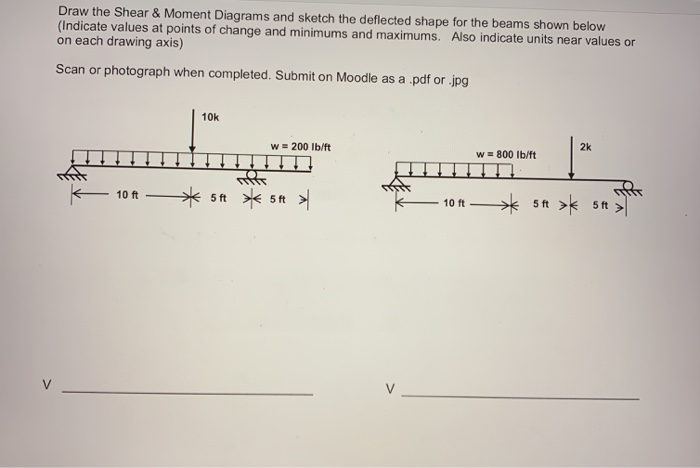Draw the Shear & Moment Diagrams and sketch the deflected shape for the beams shown below (Indicate values at points of change and minimums and maximums. Also indicate units near values or on each drawing axis) Scan or photograph when completed. Submit on Moodle as a .pdf or .jpg 10k w = 200 lb/ft 2k W = 800 lb/ft 10 ft 5 ft 5ft > 10 ft ** 5+ > 5 > M M D D

• ### Draw the shear and moment diagrams including deflected shapes. 12. 3L14 Shear Shear Moment Moment Deflected...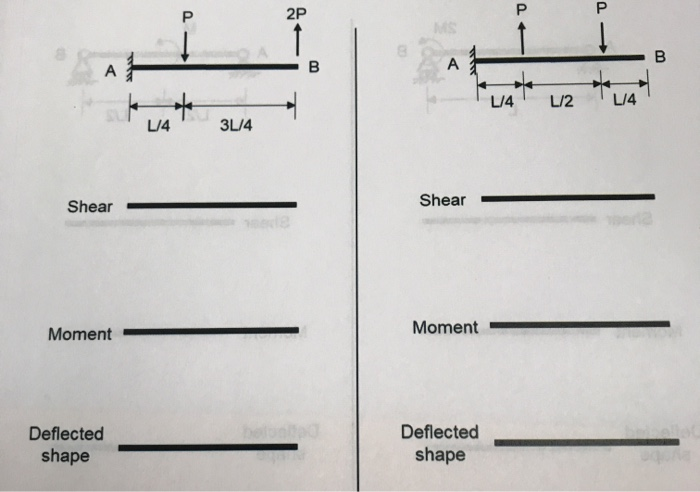Draw the shear and moment diagrams including deflected shapes. 12. 3L14 Shear Shear Moment Moment Deflected shape Deflected shape w2 12 Shear Shear Moment Moment Deflected shape Deflected shape

• ### do the 4th question plz 1-(25%) Draw shear and moment diagrams for the beam shown in Figure P-1. Draw a sketch of the deflected shape 2-(25%) Using moment area method, for the beam shown in Figure...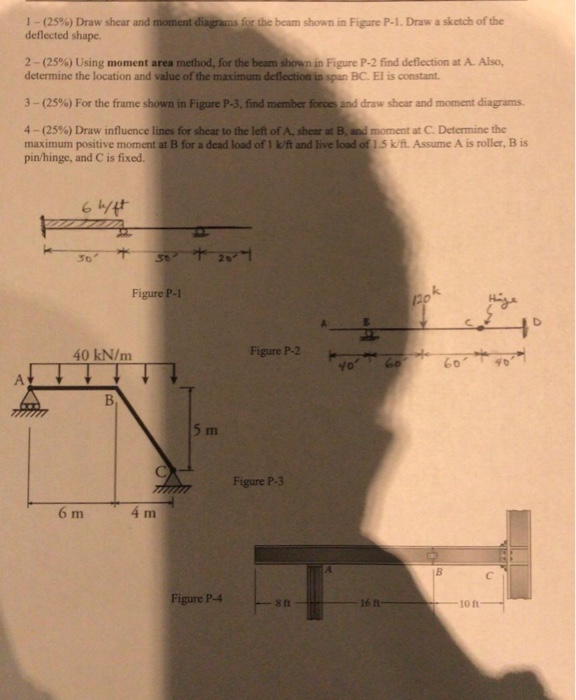do the 4th question plz 1-(25%) Draw shear and moment diagrams for the beam shown in Figure P-1. Draw a sketch of the deflected shape 2-(25%) Using moment area method, for the beam shown in Figure P-2 find deflection at A. Also, determine the location and value of the maximum deflection in span BC. El is constant 3-(25%) For the frame shown in Figure P-3-find member frees and draw shear and moment diagrams. 4-(25%) Draw/ influence lines for shear to...

• ### a Determine the reactions b) Draw the shear and moment diagrams c) Sketch the deflected shape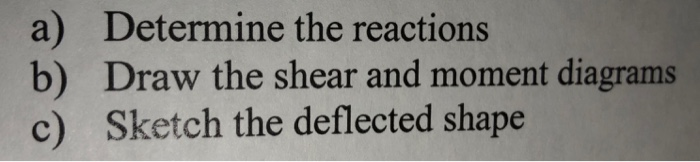a Determine the reactions b) Draw the shear and moment diagrams c) Sketch the deflected shape

• ### (40) the flexibility method down 1.5 inches and the support at D 4-Analyze the frame shown...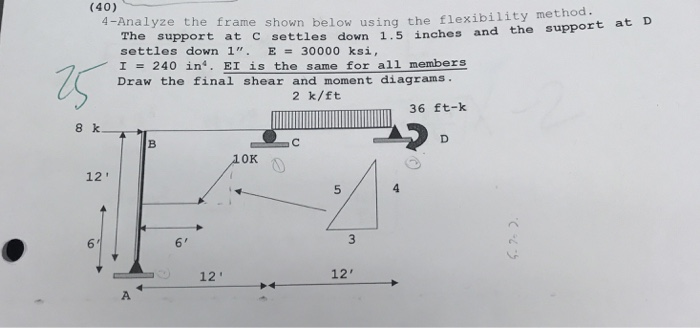(40) the flexibility method down 1.5 inches and the support at D 4-Analyze the frame shown below using The support at C settles settles down 1". E = 30000 ksi, ? 240 in4. El is the same for all members Draw the final shear and moment diagrams 2 k/ft 36 ft-k 8 k OK 12' 6 12' 12'

• ### For beam given below Draw the shear- and moment diagrams. Label all important values of shear...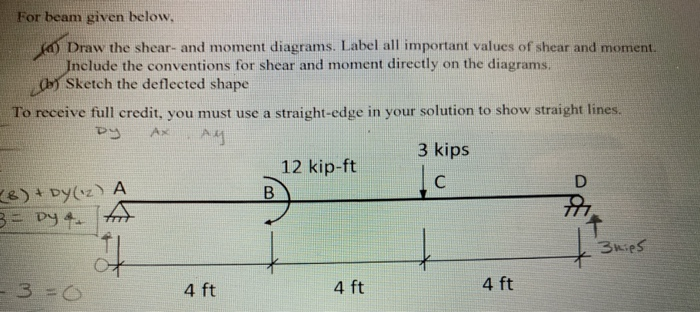For beam given below Draw the shear- and moment diagrams. Label all important values of shear and moment. nclude the conventions for shear and moment directly on the diagrams Sketch the deflected shape To reeiv leredr you mus ue astraighto sbwg ns 3 kips 12 kip-ft 4 ft 4 ft 4 ft

• ### 1. (60 points) Draw axial, shear, and bending moment diagrams for the frame shown below. Draw...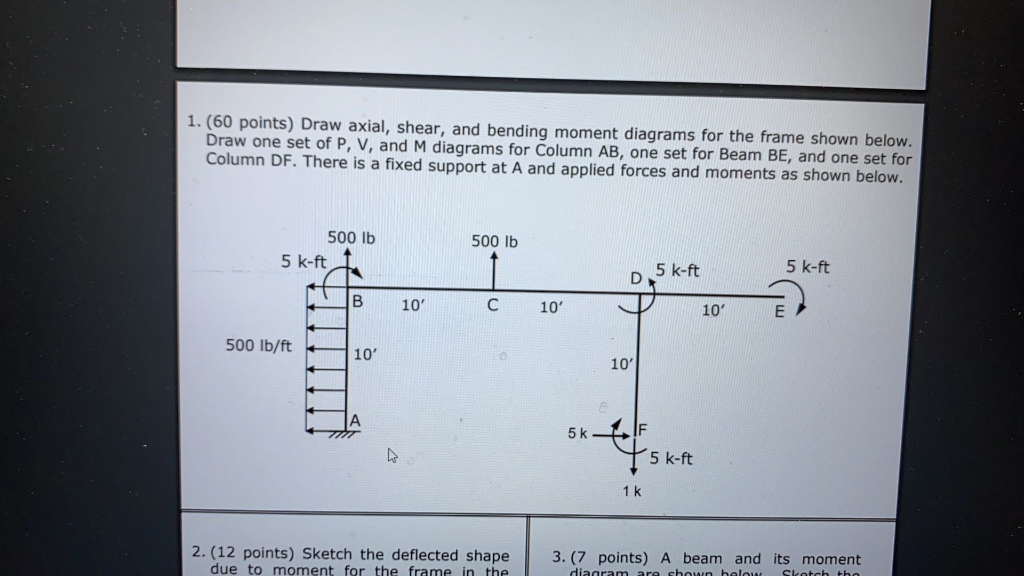1. (60 points) Draw axial, shear, and bending moment diagrams for the frame shown below. Draw one set of P, V, and M diagrams for Column AB, one set for Beam BE, and one set for Column DF. There is a fixed support at A and applied forces and moments as shown below. 500 lb 500 lb 5 k-ft 5 k-ft D 5 k-ft B 10 10' 10' E 500 lb/ft 10' 10' 5k s tiskt 5 k-ft 1k 2....

• ### 1-(25%) Draw shear and moment diagrams for the beam shown in Figure P-1 . Draw a sketch of the deflected shape. The spans are 30-ft long each (total of 90-ft), the concentrated loads are eac...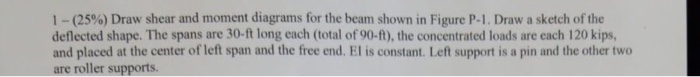1-(25%) Draw shear and moment diagrams for the beam shown in Figure P-1 . Draw a sketch of the deflected shape. The spans are 30-ft long each (total of 90-ft), the concentrated loads are each 120 kips, and placed at the center of left span and the free end. El is constant. Left support is a pin and the other two are roller supports. 120 kips 120 kips Figure P-1 1-(25%) Draw shear and moment diagrams for the beam shown...

• ### Sketch shear and moment diagrams including deflected shapes. (Indicate exact values) 2.1) PP 2PP L/2 Shear...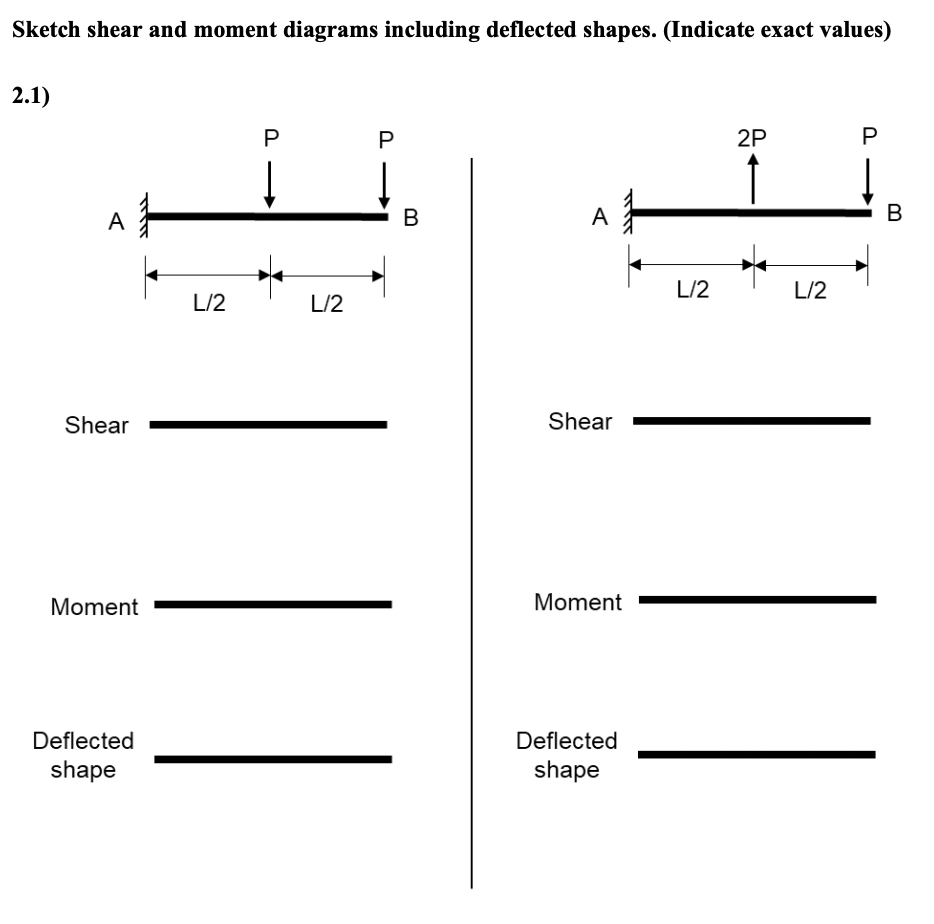Sketch shear and moment diagrams including deflected shapes. (Indicate exact values) 2.1) PP 2PP L/2 Shear Shear Moment Moment Deflected shape Deflected shape

• ### 8. Determine and Draw the quantitative shear, bending moment diagrams and qualitative deflected curve for the...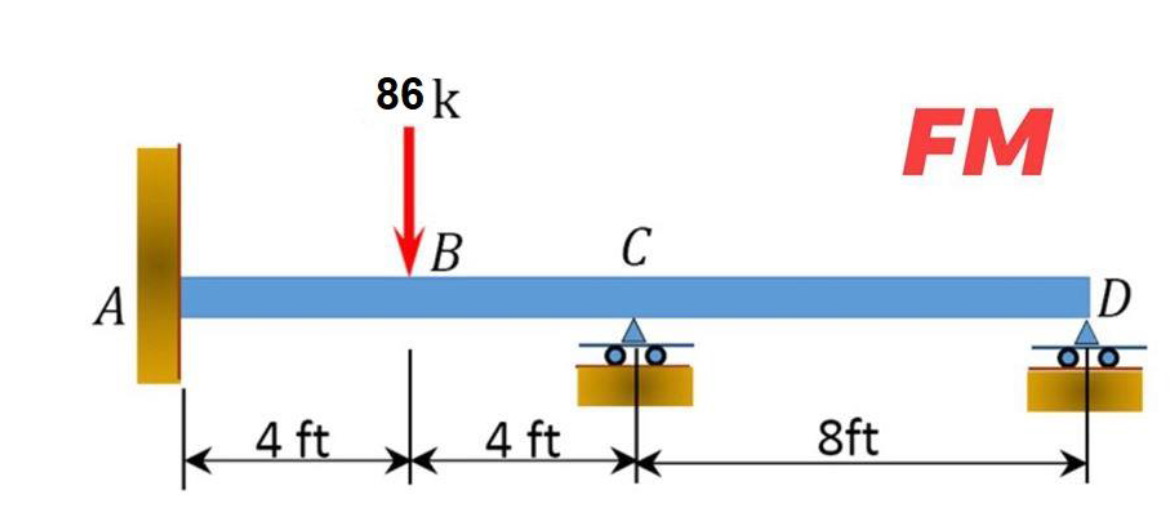8. Determine and Draw the quantitative shear, bending moment diagrams and qualitative deflected curve for the following questions by using the force method 86 k FM B C А ID ΟΙΟ. 4 ft 4 ft 8ft